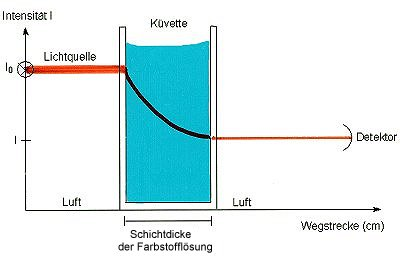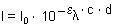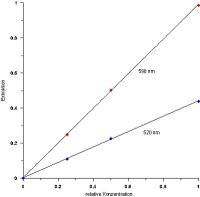# What is Lambert Beer Law in Chemistry

Experiments:
Experiment: Lambert-Beer lawWhen light travels through a colored medium, it is absorbed. Interestingly, the decrease in the intensity I of the light along the layer thickness d is described by a decay function, as we have also come to know from first-order reactions.We call the absolute power term Absorbance E. or Absorption A.

E.l = el· c · d

(Absorbance, lat. extenuo, weak.)

That expression is already that Lambert-Beer law.

In the equation mean

E.l dimensionless absorbance or absorption at a certain wavelength l
el molar extinction coefficient (cm2/ mol)
c concentration of the substance (mol / cm3)
d Layer thickness of the measuring cell (cm).

The law can also be obtained by taking the logarithm of the decay function. This makes it clear that the extinction is nothing other than the logarithmic ratio of the initial intensity I.0 to the intensity I measured after passing through the sample with the layer thickness d. This must be taken into account during the measurement. With the help of the relationship

I = I0· 10-E

we calculate the following table.

With extinctions above 2, measurement problems are already noticeable; therefore the scales on the measuring devices can no longer be read precisely here either.

The Lambert-Beer law states that the absorption of electromagnetic radiation is proportional to the concentration of the absorbing substance. It strictly only applies to visible light and UV radiation.

The molar extinction coefficient is a specific material constant; it also depends on the wavelength, since a given substance absorbs differently over the entire wavelength range, i.e. H. it shows a spectrum. This is shown in the following illustration.Spectra of crystal violet at various concentrations

The law is excellent with the triphenylmethane dye Crystal violet (KV) confirm (-> attempt).

The Lambert-Beer law is used to determine concentrations. There are two ways to do this.
First, however, a calibration curve is created in both cases by measuring the extinctions at different concentrations at a selected wavelength and plotting them against each other. This is used to check the validity of the law at all. Because you have to get a straight line. Sometimes there can be interactions with the solvent, then the straight line deviates continuously. If the measuring points in the diagram are strongly ("statistically") scattered or if a measuring point deviates significantly, errors have been made in the measurement. Examples are soiled cuvettes, formation of air bubbles in the solution, incorrect dilution measures or incorrect concentration calculations.Calibration straight line according to Lambert-Beer for crystal violet

With further absorbance measurements one can now read off the corresponding concentration values ​​graphically on the basis of this straight line.
However, one can also determine the extinction coefficient from the slope and then use this to calculate the corresponding concentration from an extinction value after converting the Lambert-Beer law. The difficulty arises that the terms of the equation are available in different dimensions!

An example
The value 1 has been measured for the extinction. The extinction coefficient is 104 cm2/ mol, the layer thickness of the cuvette is 1 cm.

c = El / (el· d)

c = 1 / (104 cm2 / mol · 1 cm)

c = 10-4 mol / cm3

To convert to mol / l we have to multiply by the factor 1000:

c = 10-1 mol / l

Further texts on the topic of reaction kinetics